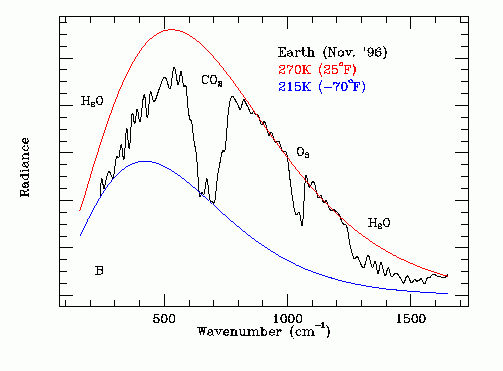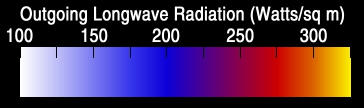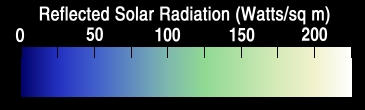# XYLENE POWER LTD.

## INFRARED EMISSION

#### By Charles Rhodes, P.Eng., Ph.D.

GLOSSARY OF TERMS

OVERVIEW OF INFRARED EMISSION:
In order to understand the infrared emission by the Earth it is necessary to understand the absorption/emission characteristics of the greenhouse gases and how these gases affect the infrared spectrum of the Earth. The gases of most interest are water vapor, carbon dioxide, ozone, methane and nitrous oxide.

At steady state conditions in any material there is a equilibrium between the rate at which electrons absorb energy from their environment and the rate at which these electrons emit photon energy. The electrons may obtain energy from either photon absorption or from changes in electron potential energy due to interactions between neighboring atoms or molecules. However, all changes in electron energy are governed by the relationship:
dE = h dF
where:
dE = photon energy;
dF = photon frequency;
h = Planck constant.

As shown on the web page titled RADIATION PHYSICS this photon energy versus frequency constraint causes the photon energy flux and the photon frequency distribution at a particular temperature within the material to adopt a Planck distribution. This photon flux and frequency distribution is apparent in the thermal radiation emitted by the material. The spectrum of the emitted radiation may be further affected by atmospheric filtering. Analysis of the emittted radiation spectrum can be used to find the material temperature at the point of photon emission and the emitted thermal radiation flux.

VIEW FROM OUTER SPACE:
When the earth is viewed from outer space with an infrared thermal emission spectrometer, the observed spectrum consists of radiation from species that will readily interact with electromagnetic radiation within the thermal emission spectral range. Oxygen and nitrogen do not have charge separation in their molecules and hence do not interact with photons in the thermal emission spectral range and hence are not seen by the space craft. Water molecules, which have charge separation, strongly interact with photons across the thermal emission spectrum. Carbon dioxide and ozone also have frequency bands of strong photon interactions.

When we look from a spacecraft at each frequency we see the temperature of the location from which those photons originate.

The water is concentrated in the lower atmosphere. Hence, from the perspective of a space craft, in the relevant frequency bands photons emitted by carbon dioxide and ozone in the upper atmosphere replace photons emitted by water in the lower atmosphere. This filtering action causes an apparent reduction in lower atmosphere photon emissivity. The photons at the point of emission have a Planck energy distribution but at the spacecraft the received photon energy distribution is altered by the by presence of the CO2 and other GHGs. In the CO2 absorption band what is seen is photons emitted by high altitude CO2 rather than photons emitted by lower altitude H2O. In the O3 absorption band what is seen is photons emitted by high altitude O3 rather than lower altitude H2O.

The H2O absorption is further frequency dependent. The H2O absorption is higher at the low frequency end of the thermal emission spectrum than in the middle of the thermal emission spectrum. Hence at the low frequency end of the thermal emission spectrum the H2O emission seen by the spacecraft is at a higher altitude than the H2O emission in the middle of the thermal emission spectrum. The temperature is lower at a higher altitude which causes an apparent reduction in emissivity as compared to the H2O emissions measured in the middle of the thermal emission spectrum.

The thermal infrared emission spectrum of the Earth, as recorded from deep space by the Mars Global Surveyor (MGS) Spacecraft in November 1996, is shown in Figure 1 below.Figure 1

THERMAL EMISSION SPECTROMETER PERFORMANCE CHECK:
Initial data from the Mars Global Surveyor Thermal Emission Spectrometer. The MGS was launched on Nov. 7 1996. The Instruments were aimed at Earth on Nov. 24th 1996 between 10-11 am MST. Scroll down the page to see the relevant text and graph. The MGS was underway for ~17 days out from launch.

THERMAL EMISSION COMPONENTS:
This recorded IR spectrum is actually the linear sum of three IR spectrums. The dominant spectrum is the IR emission from water in clouds. The phase change of water traps the emission temperature of most of the photons at 273.15 K.

In the near polar regions where the Earth surface temperature is less than 273.15 K the water content of the atmosphere is low and there is thermal emission directly from ice on or near the ground.

Near the equator where the partial pressure of water near Earth's surface is large there is thermal emission from water molecules above clouds. The temperature of these molecules is significantly less than 273.15 K.

Note that as Earth's average surface temperature increases the peak in the black body thermal emission spectrum increases in frequency, which increases the fraction of the thermal emission radiation that is blocked by atmospheric CO2. This effect amplifies the Earth surface temperature increase due to an increase in the atmospheric CO2 concentration.

ENERGY TRANSPORT THROUGH THE LOWER ATMOSPHERE:
Most of the solar energy incident on the oceans that is not reflected by clouds and ice is absorbed by sea water. This absorbed energy causes heating and hence evaporation. Most of the absorbed solar energy initially becomes latent heat of vaporization of water. The water vapor molecules rise in the atmosphere and then condense losing translational motion kinetic energy by collisions with N2 and O2 molecules. On condensation the H2O molecules clump topgether to form liquid water droplets. These liquid water droplets then cool by emission of infrared radiation and freeze. Outside of the atmospheric absorption bands the temperature of the emitted far infrared radiation photons is about 270 degrees K.

When water vapor condenses the latent heat of vaporization lost to other molecules is: 2257 J / g. The corresponding photon radiation frequencies are much higher than the thermal emission band so the primary latent heat of vaporization energy loss is via inter molecular collisions.

When liquid water cools the maximum energy loss before freezing occurs is:
(100 deg C) X (1 cal / gm deg C) X (4.18 J / cal) = 418 J / gm

The latent heat of fusion of water is:
334 J / gm

The enthalpy released in cooling ice from 0 C to - 10 C is 20.27 J / g
The enthalpy released in cooling ice from -10 C to - 20 C is 19.72 J / g
The enthalpy released in cooling ice from -20 C to - 30 C is 19.13 J / g
The enthalpy released in cooling ice from -30 C to - 40 C is 18.51 J / g
The enthalpy released in cooling ice from -40 C to - 50 C is 17.84 J / g
The enthalpy released in cooling ice from -50 C to - 58 C is 14.00 J / g

The total enthalphy release in cooling ice from 0 C to - 58 C is: 109.47 J / g

Each mole contains 6.023 X 10^23 molecules

Hence the enthalpy of fusion per molecule is:
(334 J / gm) X (18 gm H2O / mole) X (1 mole H2O / 6.023 X 10^23 molecules) = 0.998174 X 10^-20 J / molecule

Assume that liquid water freezes by emitting one infrared photon per molecule. Then when a water molecule transitions from 0 degrees C liquid to 0 degrees C solid by emitting one infrared photon the photon energy is:
E = 0.998174 X 10^-20 J

The energy of each such photon is given by:
E = h F
where h = Planck's constant
= 6.626 X 10^-34 J-s.

Hence:
F = E / h
= (0.998174 X 10^-20 J) / (6.626 X 10^-34 J-s)
= 0.150645 X 10^14 Hz
= 15.0645 THz

The speed of light is:
299,792,458 m / s

Hence the wave number of the far infrared radiation emitted by 0 degree C liquid water transitioning to 0 degree C ice is:
(Wave Number) = F / C
= (0.150645 X 10^14 Hz) / (299,792,458 m / s)
= .050249758 X 10^6 / m
= 502.5 X 10^2 / m
= 502.5 / cm

This wave number is at the peak of Earth's experimentally measured thermal infrared emission spectrum shown on Figure 1.

The wave number of the far infrared radiation emitted by 100 degree C water transitioning to freezing at -58 C is:
[(109.47 J / g + 418 J / g + 334 J / g) / (334 J / g)] (502.5 / cm) = 1296.07 / cm

This calculation explains the experimentally observed sharp drop in thermal emission at a wave number of about 1250 / cm shown in Fig.1. Note that water vapor does not emit infrared radiation at wave numbers immediately above 1300 / cm because the large heat of vaporization of water provides no accessible energy state transitions in that spectral range.

As the CO2 concentration in the Earth's upper atmosphere increases the infrared radiation emission by freezing of water droplets must increase for thermal radiation to remain in balance with absorbed solar radiation. In order to provide more warm water to drive this infrared radiation emission process the temperature of the lower atmosphere must increase.

Note that at low photon frequencies the thermal emission is attenuated by water vapor. At these frequencies the photon range is shorter than at other frequencies meaning that the photons reaching outer space originate higher in the atmosphere and hence are cooler.

CERES SATELLITE DATA
The thermal infrared emission of the Earth, as recorded from deep space by CERES Satellites is shown on the left hand side of Figure 2 below and the corresponding solar reflection is recorded on the right hand side of Fig. 2 below.Figure 2

Emission Calibration:Infrared Emission CalibrationSolar Reflection Calibration

The belt of lower IR emission near the equator is due to a high surface sea water temperature under that belt.

1. The probability of photon absorption is proportional to the number of potentially absorbing molecules in the path of the photon. This probability depends on the absorbing molecule type and the frequency of the photon. The polar nature of water molecules causes wide band IR absorption.

2.In the "water window" IR photons can travel a long distance before they are absorbed. At the edges of the "water window" that distance shortens.

3. Outside the water window IR photons are absorbed in short travel distances. Hence the temperature of photons outside the water window seen by an observer in outer space is the temperature at the top of the atmosphere.

4. The IR emission altitude is the altitude from which an emitted IR photon can escape into outer space.

5. Except near the poles almost all of Earths thermal IR photon emission into outer space is from water molecules in the atmosphere.

6. The water vapor pressure at sea level increases rapidly with increasing ocean surface water temperature.

7. The IR emission altitude increases with the water vapor pressure at sea level.

8. The IR emission temperature decreases with increasing IR emission altitude.

9. Near the equator the decrease in IR emission temperature is due to an increase in emission altitude caused by a local increase in atmospheric water vapor pressure.

10. At all latitudes the IR emission power is proportional to the fourth power of the IR emission temperature.

11. Visible clouds occur near the temperature freezing point of water.

12. Thus near the equator the IR emission photons that escape into outer space originate above the cloud layer.

13. At higher latitudes where the ocean surface temperature is less the surface water vapor pressure is less and hence the IR emission altitude falls to the altitude of the cloud layer.

14. At the poles where the surface water vapor pressure is very low the IR emission altitude falls to almost ground level.

15. On the Mars Global Surveyor data we see attenuation at low photon frequencies. This attenuation indicates that the emission altitude is weakly IR frequency dependent.

16. Over much of Earth the IR emission photons originate from latent heat of fusion of evaporated sea water. However, over the tropics the concentration of H2O molecules above the cloud layer is sufficient to reabsorb these photons so the photons that escape into outer space are cooler photons from higher in the atmosphere.

17. Over the poles where the air is very cold there is no water phase transition. The H2O molecular concentration in the atmosphere is low so the IR photons that escape into outer space originate near ground level.

18. Thus we have a qualitative explanation of all of the observed IR data.

This web page last updated November 20, 2020.